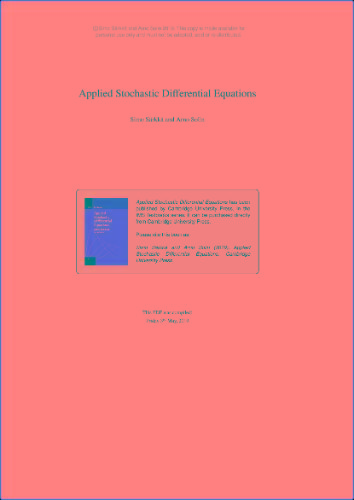## Applied Stochastic Differential EquationsApplied Stochastic Differential Equations
pdf | 3.79 MB | Author :Simo Särkkä and Arno Solin

Book Description :

Stochastic differential equations are differential equations whose solutions are stochastic processes. They exhibit appealing mathematical properties that are useful in modeling uncertainties and noisy phenomena in many disciplines. This book is motivated by applications of stochastic differential equations in target tracking and medical technology and, in particular, their use in methodologies such as filtering, smoothing, parameter estimation, and machine learning. It builds an intuitive hands-on understanding of what stochastic differential equations are all about, but also covers the essentials of Itô calculus, the central theorems in the field, and such approximation schemes as stochastic Runge-Kutta. Greater emphasis is given to solution methods than to analysis of theoretical properties of the equations. The book's practical approach assumes only prior understanding of ordinary differential equations. The numerous worked examples and end-of-chapter exercises include application-driven derivations and computational assignments. MATLAB/Octave source code is available for download, promoting hands-on work with the methods.

Category :

`https://rapidgator.net/file/88a6bced97de792600689a58f0728476/z50n5h1xs4o7.pdf`
`http://nitroflare.com/view/C7D314AB95DA9E0/7makb5c05j83.pdf`
`http://alfafile.net/file/8V9A3/z43g9rc78mi0.pdf`